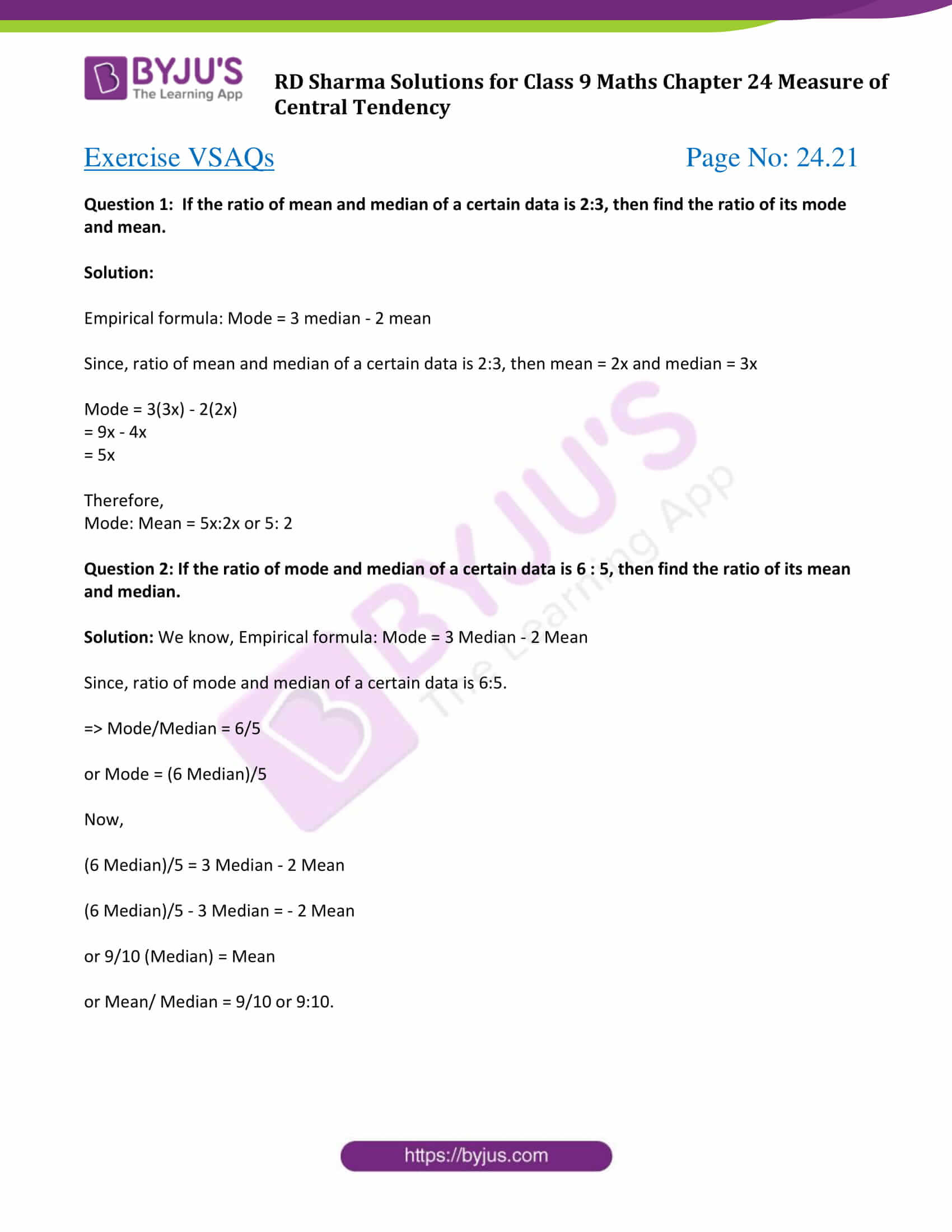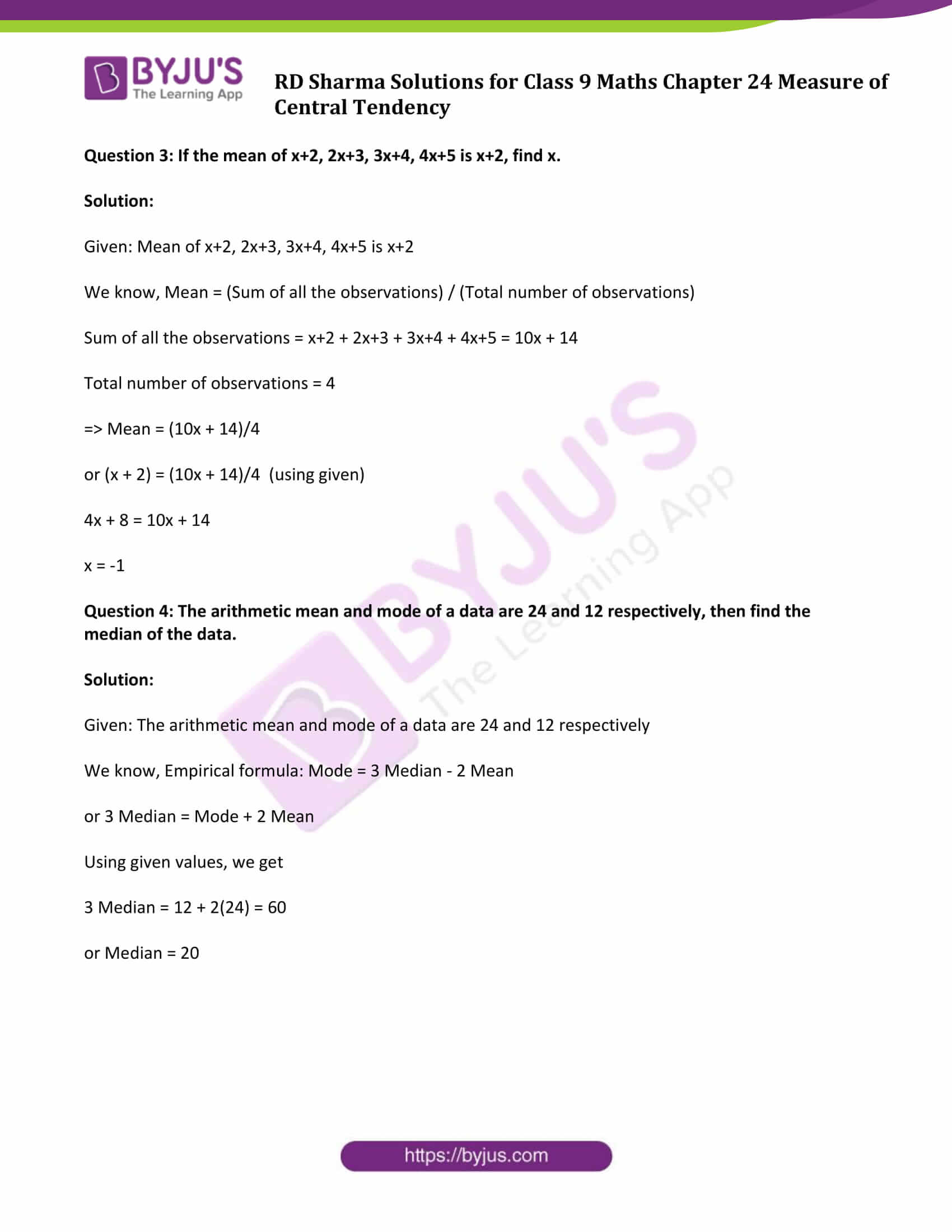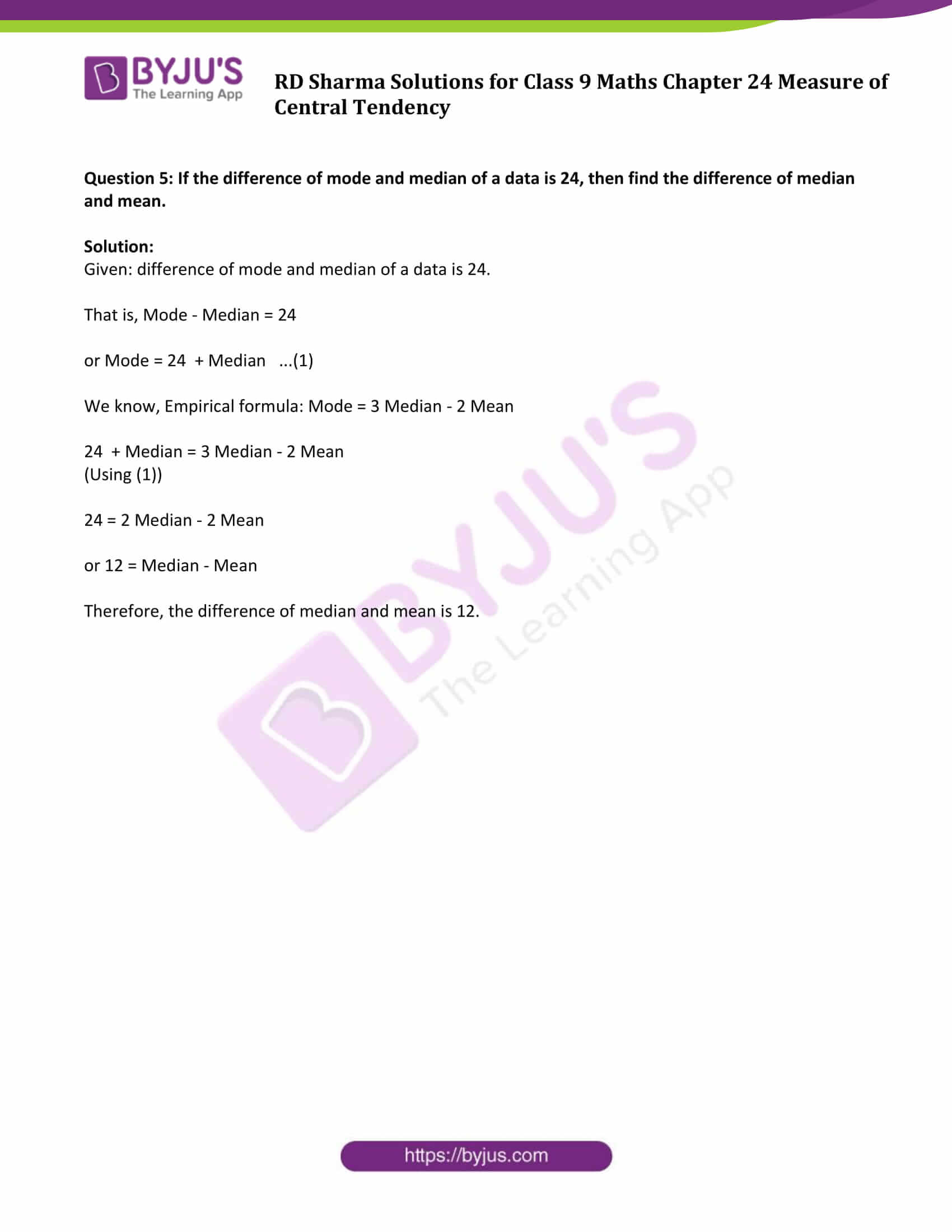# RD Sharma Solutions for Class 9 Maths Chapter 24 Measure of Central Tendency Exercise VSAQs

Class 9 Chapter 24 – Measure of Central Tendency Exercise VSAQs solutions are provided here. This exercise helps students to revise the concepts learnt in this chapter. We at BYJU’S provide RD Sharma Solutions for Class 9 Maths to assist students to comprehend all the concepts at one place. Click on the link below to access all the solutions.

## Download PDF of RD Sharma Solutions for Class 9 Maths Chapter 24 Measure of Central Tendency Exercise VSAQs### Access Answers to Maths RD Sharma Solutions for Class 9 Chapter 24 Measure of Central Tendency Exercise VSAQs Page number 24.21

Question 1: If the ratio of mean and median of a certain data is 2:3, then find the ratio of its mode and mean.

Solution:

Empirical formula: Mode = 3 median – 2 mean

Since, ratio of mean and median of a certain data is 2:3, then mean = 2x and median = 3x

Mode = 3(3x) – 2(2x)

= 9x – 4x

= 5x

Therefore,

Mode: Mean = 5x:2x or 5: 2

Question 2: If the ratio of mode and median of a certain data is 6 : 5, then find the ratio of its mean and median.

Solution: We know, Empirical formula: Mode = 3 Median – 2 Mean

Since, ratio of mode and median of a certain data is 6:5.

⇒ Mode/Median = 6/5

or Mode = (6 Median)/5

Now,

(6 Median)/5 = 3 Median – 2 Mean

(6 Median)/5 – 3 Median = – 2 Mean

or 9/10 (Median) = Mean

or Mean/ Median = 9/10 or 9:10.

Question 3: If the mean of x+2, 2x+3, 3x+4, 4x+5 is x+2, find x.

Solution:

Given: Mean of x+2, 2x+3, 3x+4, 4x+5 is x+2

We know, Mean = (Sum of all the observations) / (Total number of observations)

Sum of all the observations = x+2 + 2x+3 + 3x+4 + 4x+5 = 10x + 14

Total number of observations = 4

⇒ Mean = (10x + 14)/4

or (x + 2) = (10x + 14)/4 (using given)

4x + 8 = 10x + 14

x = -1

Question 4: The arithmetic mean and mode of a data are 24 and 12 respectively, then find the median of the data.

Solution:

Given: The arithmetic mean and mode of a data are 24 and 12 respectively

We know, Empirical formula: Mode = 3 Median – 2 Mean

or 3 Median = Mode + 2 Mean

Using given values, we get

3 Median = 12 + 2(24) = 60

or Median = 20

Question 5: If the difference of mode and median of a data is 24, then find the difference of median and mean.

Solution:

Given: difference of mode and median of a data is 24.

That is, Mode – Median = 24

or Mode = 24 + Median …(1)

We know, Empirical formula: Mode = 3 Median – 2 Mean

24 + Median = 3 Median – 2 Mean

(Using (1))

24 = 2 Median – 2 Mean

or 12 = Median – Mean

Therefore, the difference of median and mean is 12.

## RD Sharma Solutions for Class 9 Maths Chapter 24 Measures of Central Tendency Exercise VSAQs

Class 9 Maths Chapter 24 Measure of Central Tendency Exercise VSAQs is based on topics, mean, median and mode of data. This exercise covers all the basic concepts helpful to solve various statistics problems. Learning the basics is certainly necessary and the best method to study smart is by learning from RD Sharma solutions which are explained and solved chapter-wise.